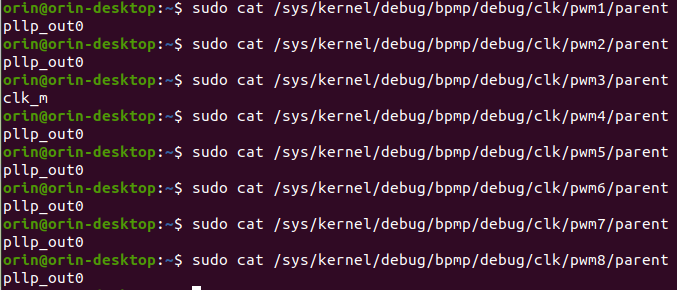# Orin PWM output is not precise to the desired PWM in code

I made a python script that uses this repo’s code: GitHub - NVIDIA/jetson-gpio: A Python library that enables the use of Jetson's GPIOs to make two of the GPIO pins (pins 15 and 18) to have two distinct PWM outputs for external sensor synchronization. I set one pin to be 50Hz and the other to be 400Hz using GPIO.PWM(). I gave each with a duty cycle of 10% and hooked up an oscilloscope to verify the outputs. Strangely I found that while the 50Hz signal was very close (~49.99043 to 50.00511Hz) the 400Hz signal was off by a few HZ (~401.5 to 402.0Hz). I also noted that this range is the same when I set the frequency to anywhere between 396 and 408 Hz (13Hz range). Is there any explanation to why this happens, or any way to make the PWM more precise?

I looked at section 5.11 in the data sheet and saw that there is two clocks (OSC and PLLP) is this somewhat related?

I also have tried both switching the oscilloscope probes and which pins are outputting which frequency. The result is still the same.

Any help is appreciated! Thanks!

Oscilloscope reading picture (sorry for the glare):

Sorry for the late response, our team will do the investigation and provide suggestions soon. Thanks

hello jason.heng,

could you please examine debugfs for PWM setting.
for example,

``````Check possible clock parents of pwmN.
\$ cat /sys/kernel/debug/bpmp/debug/clk/pwm8/possible_parents
Current rate of pwmN (in Hz):
\$ cat /sys/kernel/debug/bpmp/debug/clk/pwmN/rate
Set a new source from possible sources
\$ echo <new_source> > /sys/kernel/debug/bpmp/debug/clk/pwmN/parent
``````

here also given an example to calculate possible rate of a PWM controller.
assume clock source as `pllp_out0` which has a rate of 102 MHz.
hence…
Max Rate = `102Mhz/ 256 = 398437 Hz = 2510 ns`
Min Rate = `102Mhz/ (256* 2^13) (i.e. 13-bits Frequency divider (bits 0:12)) = 48.63Hz = 20561324 ns`

you shall configure PWM periods / duty_cycle within that range,

3187500 / 256 = 12451.17188
3187500 / (256 * 2^13) = 1.519918442
136000000 / 256 = 531250
136000000 / (256 * 2^13) = 64.84985352
4800000 / 256 = 18750
4800000 / (256 * 2^13) = 2.288818359
408000000 / 256 = 1593750
408000000 / (256 * 2^13) = 194.5495605

The 400Hz I’m trying to acquire is between the values of all the channels. So I’m pretty sure it being out of range isn’t the issue.I’m not sure which PWM number corresponds to which pins (I didn’t see any specifications of what PWM corresponds to what pin in the data sheet) so I tried changing the parent of each of the PWMs but I saw no changes in my output square wave (It was still ~401.5 to 402.0Hz instead of 400Hz).

I’m fairly new to using the Orin still so I might have missed an important detail.